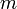# Difference between revisions of "FAQ: What does it mean for a non-square matrix to be full rank?"

Jump to: navigation, search

(Contributed by Richard Murray, 17 May 2008)

A matrix is full row rank when each of the rows of the matrix are linearly independent and full column rank when each of the columns of the matrix are linearly independent. For a square matrix these two concepts are equivalent and we say the matrix is full rank if all rows and columns are linearly independent. A square matrix is full rank if and only if its determinant is nonzero.

For a non-square matrix withrows andcolumns, it will always be the case that either the rows or columns (whichever is larger in number) are linearly independent. Hence when we say that a non-square matrix is full rank, we mean that the row and column rank are as high as possible, given the shape of the matrix. So if there are more rows than columns (), then the matrix is full rank if the matrix is full column rank.

### Further reading

• G. Strang. Linear Algebra and Its Applications, 3rd ed. Harcourt Brace Jovanovich, San Diego, 1988.
• Wikipedia: Rank (linear algebra)